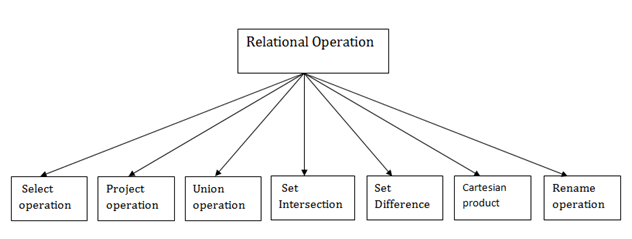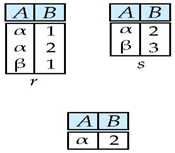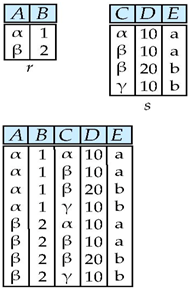## Relational algebra operations

Relational Algebra is procedural query languages, which takes Relation as input and generate relation as output. Relational algebra mainly provides theoretical foundation for relational databases and SQL.Selection (σ)

Selection is used to select required tuples of the relations.

Projection (π) :Projection is used to project required column data from a relation.

By Default projection removes duplicate data.

Union (U)

Union operation in relational algebra is same as union operation in set theory, only constraint is for union of two relations both relations must have same set of Attributes

Set Difference (-)

Set Difference in relational algebra is same set difference operation as in set theory with the constraint that both relations should have same set of attributes.

Set Intersection:

Suppose there are two tuples R and S. The set intersection operation contains all tuples that are in both R & S. It is denoted by intersection ∩.Cross Product (X)

Cross product between two relations let say R and S, so cross product between R X S will results all the attributes of R followed by each attribute of S. Each record of R will pairs with every record of SRename (ρ)

Rename is a unary operation used for renaming attributes of a relation. ρ (a/b)R will rename the attribute ‘b’ of relation by ‘a’.

Note: only a member of this blog may post a comment.

More

More

More

More

More

More
Top